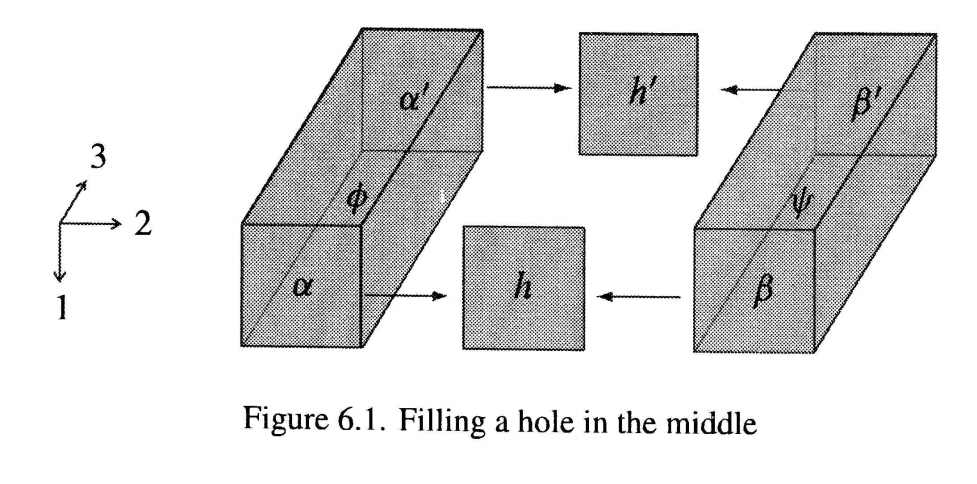# $2$-Morphisms in the Fundamental $2$-Groupoid

I'm trying to write down a clean definition of the fundamental $2$-groupoid $\pi_{\leq 2}(X)$ of a topological space $X$. Specifically, I'm concerned with how to properly define $2$-morphisms. Here is what I have:

The fundamental $2$-groupoid $\pi_{\leq 2}(X)$ is the strict $2$-category where

• The objects of $\pi_{\leq 2}(X)$ are the points of $X$.
• The $1$-morphisms $x\rightarrow y$ of $\pi_{\leq 2}(X)$ are the continuous maps $f:[0,|f|]\rightarrow X$ with $f(0)=x$ and $f(|f|)=y$. Here $|f|\geq 0$.
• For $x\xrightarrow{f}y\xrightarrow{g}z$ define $g\circ f$ as the continuous map $[0,|f|+|g|]\rightarrow X$ given by $$(g\circ f)(t) = \begin{cases} f(t) & 0\leq t\leq|f| \\ g(t-|f|) & |f|\leq t\leq|f|+|g| \end{cases}$$

Of course, we are deviating from the usual definition of a path as a map $[0,1]\rightarrow X$ to make the composition of $1$-morphisms associative on the nose. However, this seems to create a new problem. The $2$-morphisms should be homotopies of paths, but a homotopy between two maps is only defined if the maps have common domain and codomain. To remedy the situation, I've tried the following:

• For $x\xrightarrow{f}y$, let $\overline{f}:x\rightarrow y$ be the continuous map $[0,1]\rightarrow X$ given by $\overline{f}(t)=f(|f|\cdot t)$. Then the $2$-morphisms $\alpha:f\Rightarrow g$ in $\pi_{\leq 2}(X)$ are the continuous maps $\alpha:[0,1]\times[0,1]\rightarrow X$ with $\alpha(t,0)=\overline{f}(t)$, $\alpha(t,1)=\overline{g}(t)$, $\alpha(0,t)=x$, and $\alpha(1,t)=y$.

Is this the standard construction? I'm having trouble finding literature on this.

• Don't forget that, because you are truncating at $2$, your $2$-morphisms shouldn't be maps, but instead be homotopy equivalence classes of maps. – user14972 Jan 29 '14 at 5:23
• Oh right. So is my definition correct, up to homotopy? – Brian Fitzpatrick Jan 29 '14 at 5:25
• Probably, but I have little experience so I'll leave it for someone else to answer. Incidentally, if I was taking your approach for the $1$-morphisms, I probably would have defined homotopies to be maps from the relevant trapezoids rather than from squares. Of course, that makes composition annoying. :( – user14972 Jan 29 '14 at 5:26
• ... and then I'd probably decide that the domains should be a homotopy type, then get annoyed the construction is getting complicated, then go back to squares rather than deal with it. :( – user14972 Jan 29 '14 at 5:30
• You meet try working with Moore rectangles: see arXiv:0909.2212 Moore hyperrectangles on a space form a strict cubical omega-category. But in the context of spaces, and no extra structure, there will always be problems with getting a strict 2-groupoid or double groupoid, in my view. – Ronnie Brown Feb 2 '14 at 23:10

This is a question which raises all sorts of interesting issues!

I think it is easier to look for double groupoids rather than $2$-groupoids, because it is more symmetric with regard to directions and before taking homotopy classes of some kind, the notion of partial composition in a given direction is very clear, and extends to all dimensions. See also this question on mathoverflow.

I though about this for 9 years starting in 1965 with the aim, as it seemed likely that the proof I had written out many times for my topology book extended to get a $2$-dimensional van Kampen type theorem, and got myself quite confused. So it was "an idea for a proof in search of a theorem".

The breakthrough came with Philip Higgins in 1974 when, as suggested by work of J H C Whitehead on second relative homotopy groups, we started looking not at spaces but at pairs of spaces, $(X,A)$. So the natural idea was to look at maps of square $I^2$ into $X$ which took the vertices to the base point and edges into $A$. Then all became easy, not trivial, but easy. The details are in this book, which has a free pdf. A key picture for the definition of compositions being well defined isThe point is that that if $\alpha, \beta$ are two squares as above then their classes $[\alpha], [\beta]$ are homotopy classes rel vertices of maps $I^2 \to X$ where during the homotopies the boundary of $I^2$ stays in $A$. So $\partial^+_2[\alpha] =\partial^2_-[\beta]$ means there is a homotopy $h$ of two loops in $A$, and such a homotopy is arbitrary. So when you try to prove independence of choices, you get the above figure, which has a big hole in the middle! But the bottom edges of the hole are all constant, so you can fill in the bottom square. Now fill in the hole by a retraction. All the faces of the "hole" are in $A$ so in the retraction the top face is also in $A$. So the whole block gives a homotopy $$\alpha +_2 h +_2 \beta \simeq \alpha' +_2 h'+_2 \beta'$$ of the type required.

This construction we called $\rho_2(X,A,a)$. It contains $\pi_2(X,A,a)$ . From this you can get as a substructure a $2$-groupoid, if you really want it.

There are papers dealing with the absolute case for Hausdorff spaces, see K.A. Hardie, K.H. Kamps and R.W. Kieboom, ‘A homotopy 2–groupoid of a Hausdorﬀ space’, Applied Cat. Structures 8 (2000), 209–234, and subsequent papers dealing with double groupoids in a related fashion. But these papers have not it seems led to new calculations of homotopical invariants, as has the above relative construction.

Edit Feb 9, 2014: The above construction of a homotopy double groupoid of a pair is used to prove a $2$-dimensional van Kampen type theorem, one of whose consequences, an excision type theorem for second relative homotopy groups, is explained in my answer to this stackexchange question.